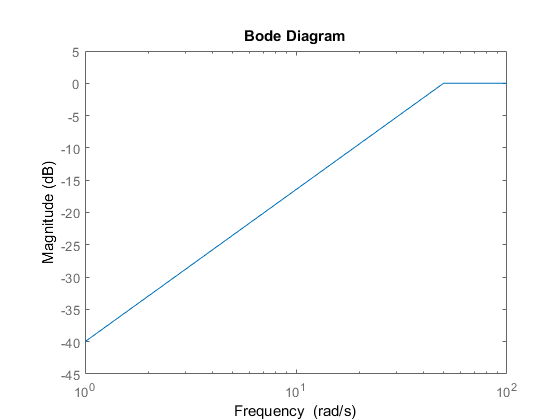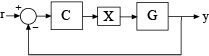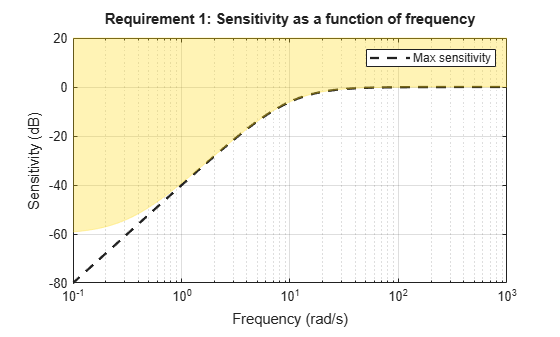# TuningGoal.Sensitivity class

Package: TuningGoal

Sensitivity requirement for control system tuning

## Description

Use `TuningGoal.Sensitivity` to limit the sensitivity of a feedback loop to disturbances. Constrain the sensitivity to be smaller than one at frequencies where you need good disturbance rejection. Use this tuning goal for control system tuning with tuning commands such as `systune` or `looptune`.

## Construction

`Req = TuningGoal.Sensitivity(location,maxsens)` creates a tuning goal for limiting the sensitivity to disturbances entering a feedback loop at the specified location. `maxsens` specifies the maximum sensitivity as a function of frequency. You can specify the maximum sensitivity profile as a smooth transfer function or sketch a piecewise error profile using an `frd` model or the `makeweight` (Robust Control Toolbox) command.

See `getSensitivity` (Simulink Control Design) for more information about sensitivity functions.)

### Input Arguments

 `location` Location at which the sensitivity to disturbances is constrained, specified as a character vector or cell array of character vectors that identify one or more locations in the control system to tune. What locations are available depends on what kind of system you are tuning:If you are tuning a Simulink® model of a control system, you can use any linear analysis point marked in the model, or any linear analysis point in an `slTuner` (Simulink Control Design) interface associated with the Simulink model. Use `addPoint` (Simulink Control Design) to add analysis points to the `slTuner` interface. For example, if the `slTuner` interface contains an analysis point `u`, you can use `'u'` to refer to that point when creating tuning goals. Use `getPoints` (Simulink Control Design) to get the list of analysis points available in an `slTuner` interface to your model.If you are tuning a generalized state-space (`genss`) model of a control system, you can use any `AnalysisPoint` location in the control system model. For example, the following code creates a PI loop with an analysis point at the plant input `'u'`. ```AP = AnalysisPoint('u'); G = tf(1,[1 2]); C = tunablePID('C','pi'); T = feedback(G*AP*C,1); ```When creating tuning goals, you can use `'u'` to refer to the analysis point at the plant input. Use `getPoints` to get the list of analysis points available in a `genss` model. If `location` is a cell array, then the sensitivity requirement applies to the MIMO loop. `maxsens` Maximum sensitivity to disturbances as a function of frequency. You can specify `maxsens` as a smooth SISO transfer function (`tf`, `zpk`, or `ss` model). Alternatively, you can sketch a piecewise gain profile using a `frd` model or the `makeweight` (Robust Control Toolbox) command. For example, the following `frd` model specifies a maximum sensitivity of 0.01 (–40 dB) at 1 rad/s, increasing to 1 (0 dB) past 50 rad/s. ```maxsens = frd([0.01 1 1],[1 50 100]); bodemag(maxsens) ylim([-45,5])```When you use an `frd` model to specify `maxsens`, the software automatically maps your specified gain profile to a `zpk` model whose magnitude approximates the desired gain profile. Use `viewGoal(Req)` to plot the magnitude of that `zpk` model. If you are tuning in discrete time (that is, using a `genss` model or `slTuner` interface with nonzero `Ts`), you can specify `maxsens` as a discrete-time model with the same `Ts`. If you specify `maxsens` in continuous time, the tuning software discretizes it. Specifying the maximum sensitivity profile in discrete time gives you more control over the profile near the Nyquist frequency.

## Properties

 `MaxSensitivity` Maximum sensitivity as a function of frequency, specified as a SISO `zpk` model. The software automatically maps the input argument `maxsens` onto a `zpk` model. The magnitude of this `zpk` model approximates the desired gain profile. Use `viewGoal(Req)` to plot the magnitude of the `zpk` model `MaxSensitivity`. `Focus` Frequency band in which tuning goal is enforced, specified as a row vector of the form `[min,max]`. Set the `Focus` property to limit enforcement of the tuning goal to a particular frequency band. Express this value in the frequency units of the control system model you are tuning (rad/`TimeUnit`). For example, suppose `Req` is a tuning goal that you want to apply only between 1 and 100 rad/s. To restrict the tuning goal to this band, use the following command:`Req.Focus = [1,100];` Default: `[0,Inf]` for continuous time; `[0,pi/Ts]` for discrete time, where `Ts` is the model sample time. `LoopScaling` Toggle for automatically scaling loop signals, specified as `'on'` or `'off'`. In multi-loop or MIMO control systems, the feedback channels are automatically rescaled to equalize the off-diagonal terms in the open-loop transfer function (loop interaction terms). Set `LoopScaling` to `'off'` to disable such scaling and shape the unscaled sensitivity function. Default: `'on'` `Location` Location of disturbance, specified as a cell array of character vectors that identify one or more analysis points in the control system to tune. For example, if `Location = {'u'}`, the tuning goal evaluates the open-loop response measured at an analysis point `'u'`. If `Location = {'u1','u2'}`, the tuning goal evaluates the MIMO open-loop response measured at analysis points `'u1'` and `'u2'`. The initial value of the `Location` property is set by the `location` input argument when you create the tuning goal. `Models` Models to which the tuning goal applies, specified as a vector of indices. Use the `Models` property when tuning an array of control system models with `systune`, to enforce a tuning goal for a subset of models in the array. For example, suppose you want to apply the tuning goal, `Req`, to the second, third, and fourth models in a model array passed to `systune`. To restrict enforcement of the tuning goal, use the following command: `Req.Models = 2:4;` When `Models = NaN`, the tuning goal applies to all models. Default: `NaN` `Openings` Feedback loops to open when evaluating the tuning goal, specified as a cell array of character vectors that identify loop-opening locations. The tuning goal is evaluated against the open-loop configuration created by opening feedback loops at the locations you identify. If you are using the tuning goal to tune a Simulink model of a control system, then `Openings` can include any linear analysis point marked in the model, or any linear analysis point in an `slTuner` (Simulink Control Design) interface associated with the Simulink model. Use `addPoint` (Simulink Control Design) to add analysis points and loop openings to the `slTuner` interface. Use `getPoints` (Simulink Control Design) to get the list of analysis points available in an `slTuner` interface to your model. If you are using the tuning goal to tune a generalized state-space (`genss`) model of a control system, then `Openings` can include any `AnalysisPoint` location in the control system model. Use `getPoints` to get the list of analysis points available in the `genss` model. For example, if `Openings = {'u1','u2'}`, then the tuning goal is evaluated with loops open at analysis points `u1` and `u2`. Default: `{}` `Name` Name of the tuning goal, specified as a character vector. For example, if `Req` is a tuning goal: `Req.Name = 'LoopReq';` Default: `[]`

## Examples

### Disturbance Sensitivity at Plant Input

Create a tuning goal that limits the sensitivity to disturbance at the plant input of the following control system. The control system contains an analysis point named `'X'` at the plant input.Specify a maximum sensitivity of 0.01 (–40 dB) at 1 rad/s, increasing to 1 (0 dB) past 10 rad/s. Use an `frd` model to sketch this target sensitivity.

```maxsens = frd([0.01 1 1],[1 10 100]); Req = TuningGoal.Sensitivity('X',maxsens);```

The software converts `maxsens` into a smooth function of frequency that approximates the piecewise-specified gain profile. Visualize this function using `viewGoal`.

`viewGoal(Req)`The shaded region indicates regions where the tuning goal is violated. The shaded region differs from the specified gain profile (dashed line) at very low frequencies because of modifications that the software introduces for numeric stability, as described in Algorithms.

### Sensitivity Goal with Limited Frequency Range and Model Application

Create a tuning goal that specifies a maximum sensitivity of 0.1 (10%) at frequencies below 5 rad/s. Configure the tuning goal to apply only to the second and third plant models.

```Req = TuningGoal.Sensitivity('u',0.1); Req.Focus = [0 5]; Req.Models = [2 3];```

You can use `Req` as an input to `looptune` or `systune` when tuning a control system that has an analysis point called `'u'`. Setting the `Focus` property limits the application of the tuning goal to frequencies between 0 and 5 rad/s. Setting the `Models` property restricts application of the tuning goal to the second and third models in an array, when you use the tuning goal to tune an array of control system models.

## Tips

• This tuning goal imposes an implicit stability constraint on the closed-loop sensitivity function measured at `Location`, evaluated with loops opened at the points identified in `Openings`. The dynamics affected by this implicit constraint are the stabilized dynamics for this tuning goal. The `MinDecay` and `MaxRadius` options of `systuneOptions` control the bounds on these implicitly constrained dynamics. If the optimization fails to meet the default bounds, or if the default bounds conflict with other requirements, use `systuneOptions` to change these defaults.

## Algorithms

When you tune a control system using a `TuningGoal`, the software converts the tuning goal into a normalized scalar value f(x), where x is the vector of free (tunable) parameters in the control system. The software then adjusts the parameter values to minimize f(x) or to drive f(x) below 1 if the tuning goal is a hard constraint.

For `TuningGoal.Sensitivity`, f(x) is given by:

`$f\left(x\right)={‖{W}_{S}\left(s\right)S\left(s,x\right)‖}_{\infty },$`

or its discrete-time equivalent. Here, S(s,x) is the closed-loop sensitivity function measured at the location specified in the tuning goal. WS is a frequency weighting function derived from the specified sensitivity profile. The gains of WS and `1/MaxSensitivity` roughly match for gain values ranging from –20 dB to 60 dB. For numerical reasons, the weighting function levels off outside this range, unless the specified sensitivity profile changes slope outside this range. This adjustment is called regularization. Because poles of WS close to s = 0 or s = `Inf` might lead to poor numeric conditioning of the `systune` optimization problem, it is not recommended to specify sensitivity profiles with very low-frequency or very high-frequency dynamics.

To obtain WS, use:

`WS = getWeight(Req,Ts)`

where `Req` is the tuning goal, and `Ts` is the sample time at which you are tuning (`Ts = 0` for continuous time). For more information about regularization and its effects, see Visualize Tuning Goals.

## Compatibility Considerations

expand all

Behavior changed in R2016a

Introduced in R2016a